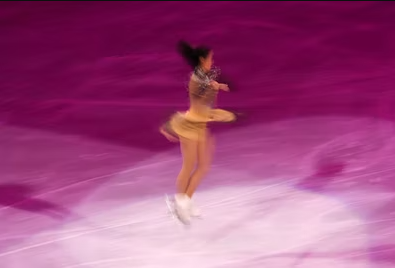## Figure Skating

Consider a lone Olympic figure skater is performing a spinning trick on ice. If she wanted to rotate faster mid spin, should she extend her arms outwards or inwards? Why?Hint
The problem is asking how to increase angular velocity:
$$\omega=\omega_0+\alpha t$$$where $$\omega_0$$ is initial angular velocity, $$\alpha$$ is angular acceleration, and $$t$$ is time. Hint 2 Because moving the skater’s arms inwards or outwards doesn’t affect initial angular velocity or time, $$\omega_0$$ and $$t$$ can be ignored as constant values. Therefore, angular acceleration is the variable we need to focus on. For rotation about some arbitrary fixed axis $$q$$ : $$\sum M_q =I_q\alpha$$$
where $$M_q$$ is the moment about axis $$q$$ , and $$I_q$$ is the mass moment of inertia.
The problem is asking how to increase angular velocity:
$$\omega=\omega_0+\alpha t$$$where $$\omega_0$$ is initial angular velocity, $$\alpha$$ is angular acceleration, and $$t$$ is time. Because moving the skater’s arms inwards or outwards doesn’t affect initial angular velocity or time, $$\omega_0$$ and $$t$$ can be ignored as constant values. Therefore, angular acceleration is the variable we need to focus on. For rotation about some arbitrary fixed axis $$q$$ : $$\sum M_q =I_q\alpha$$$
where $$M_q$$ is the applied moment about axis $$q$$ (which is also known as angular moment/movement around an axis), and $$I_q$$ is the mass moment of inertia (which describes how the body/mass is distributed relative to the spin axis).
Rearranging the equation:
$$\alpha=\frac{M_q}{I_q}$$$To increase angular velocity, angular acceleration must increase. Based on the above equation, angular acceleration increases when the moment magnifies or when the mass moment of inertia drops. If we simplify the figure skater to a point mass, the general form for the mass moment of inertia is: $$I=mr^2$$$
where $$m$$ is the mass, and $$r$$ is the perpendicular distance to the rotation axis, $$q$$ .
By moving her arms inward, the figure skater is reducing the body’s perpendicular distance from the center rotation axis. This decreases her mass moment of inertia and increases her rotating speed. The opposite would happen if she were to extend her arms outward.
Inwards due to decreased moment of inertia.